# Lecture 6_Panel Data Models.pdf

2 Jun 2023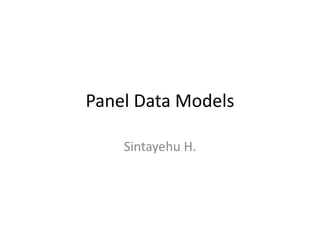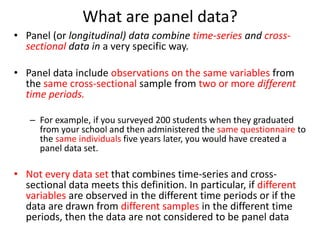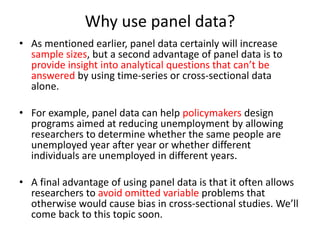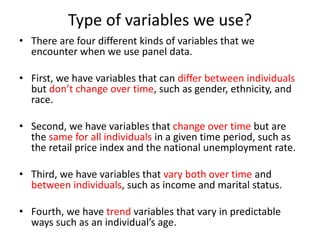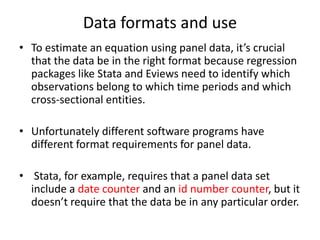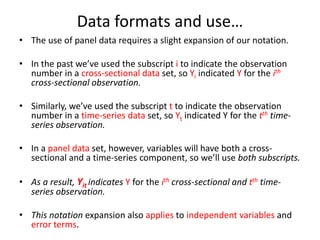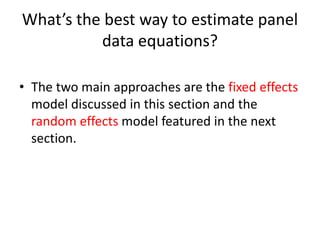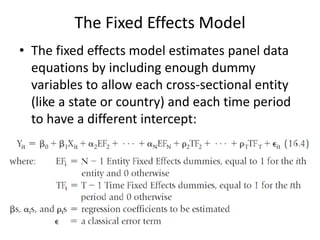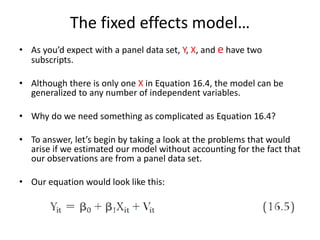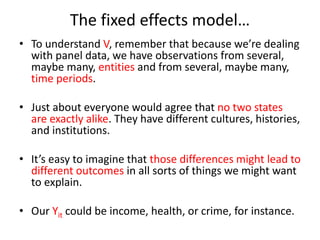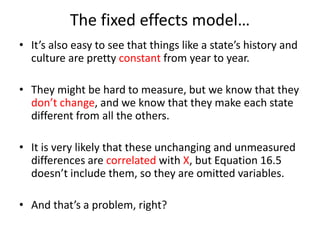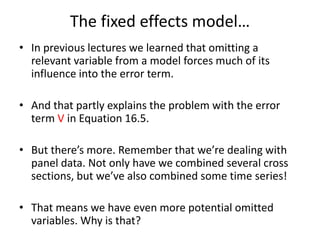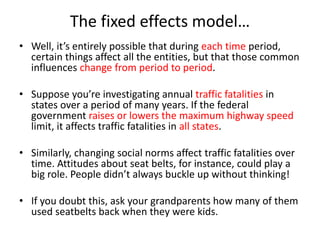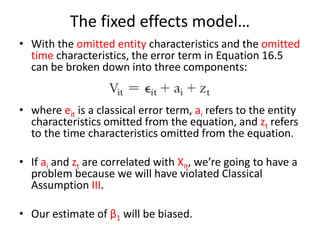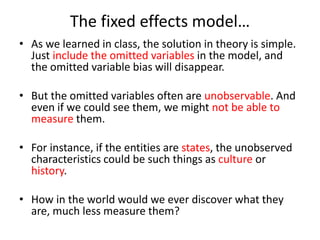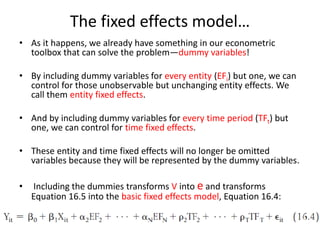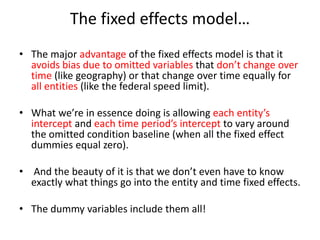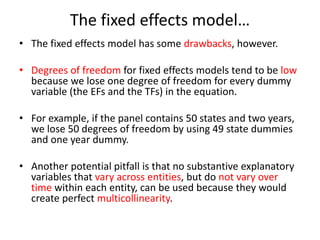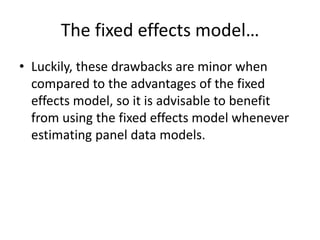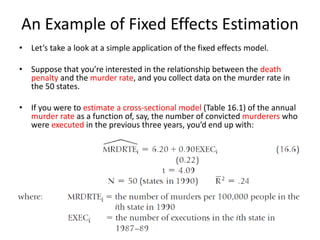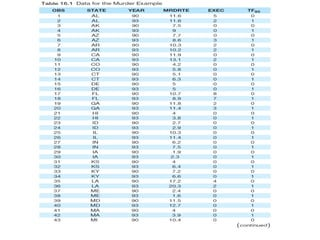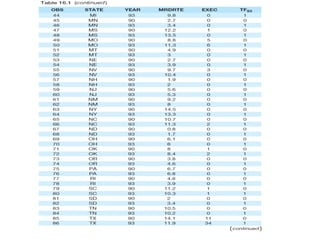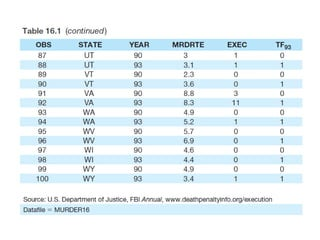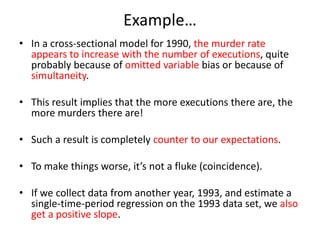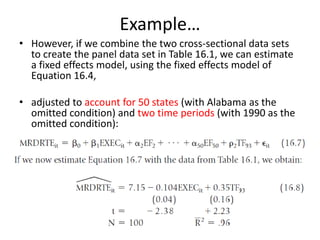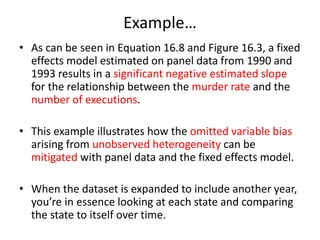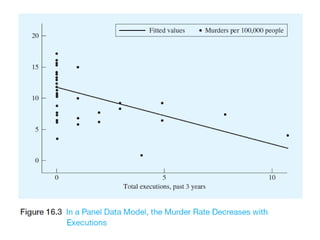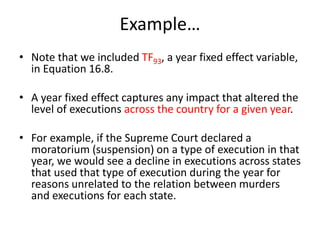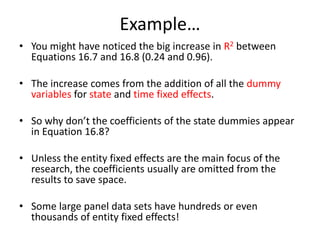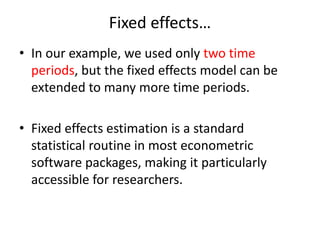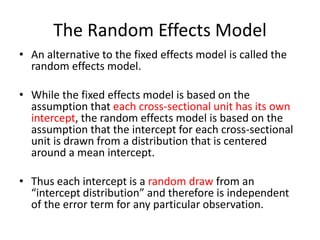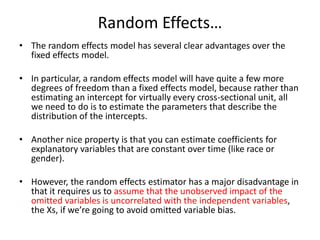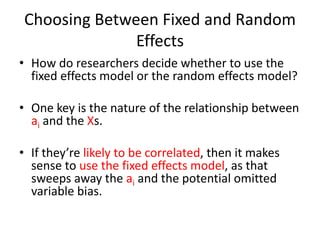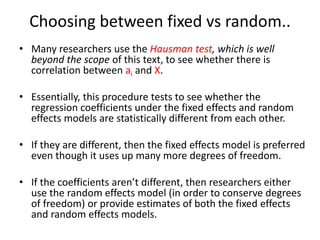1 sur 35

### Lecture 6_Panel Data Models.pdf

• 1. Panel Data Models Sintayehu H.
• 2. What are panel data? • Panel (or longitudinal) data combine time-series and cross- sectional data in a very specific way. • Panel data include observations on the same variables from the same cross-sectional sample from two or more different time periods. – For example, if you surveyed 200 students when they graduated from your school and then administered the same questionnaire to the same individuals five years later, you would have created a panel data set. • Not every data set that combines time-series and cross- sectional data meets this definition. In particular, if different variables are observed in the different time periods or if the data are drawn from different samples in the different time periods, then the data are not considered to be panel data
• 3. Why use panel data? • As mentioned earlier, panel data certainly will increase sample sizes, but a second advantage of panel data is to provide insight into analytical questions that can’t be answered by using time-series or cross-sectional data alone. • For example, panel data can help policymakers design programs aimed at reducing unemployment by allowing researchers to determine whether the same people are unemployed year after year or whether different individuals are unemployed in different years. • A final advantage of using panel data is that it often allows researchers to avoid omitted variable problems that otherwise would cause bias in cross-sectional studies. We’ll come back to this topic soon.
• 4. Type of variables we use? • There are four different kinds of variables that we encounter when we use panel data. • First, we have variables that can differ between individuals but don’t change over time, such as gender, ethnicity, and race. • Second, we have variables that change over time but are the same for all individuals in a given time period, such as the retail price index and the national unemployment rate. • Third, we have variables that vary both over time and between individuals, such as income and marital status. • Fourth, we have trend variables that vary in predictable ways such as an individual’s age.
• 5. Data formats and use • To estimate an equation using panel data, it’s crucial that the data be in the right format because regression packages like Stata and Eviews need to identify which observations belong to which time periods and which cross-sectional entities. • Unfortunately different software programs have different format requirements for panel data. • Stata, for example, requires that a panel data set include a date counter and an id number counter, but it doesn’t require that the data be in any particular order.
• 6. Data formats and use… • The use of panel data requires a slight expansion of our notation. • In the past we’ve used the subscript i to indicate the observation number in a cross-sectional data set, so Yi indicated Y for the ith cross-sectional observation. • Similarly, we’ve used the subscript t to indicate the observation number in a time-series data set, so Yt indicated Y for the tth time- series observation. • In a panel data set, however, variables will have both a cross- sectional and a time-series component, so we’ll use both subscripts. • As a result, Yit indicates Y for the ith cross-sectional and tth time- series observation. • This notation expansion also applies to independent variables and error terms.
• 7. What’s the best way to estimate panel data equations? • The two main approaches are the fixed effects model discussed in this section and the random effects model featured in the next section.
• 8. The Fixed Effects Model • The fixed effects model estimates panel data equations by including enough dummy variables to allow each cross-sectional entity (like a state or country) and each time period to have a different intercept:
• 9. The fixed effects model… • As you’d expect with a panel data set, Y, X, and e have two subscripts. • Although there is only one X in Equation 16.4, the model can be generalized to any number of independent variables. • Why do we need something as complicated as Equation 16.4? • To answer, let’s begin by taking a look at the problems that would arise if we estimated our model without accounting for the fact that our observations are from a panel data set. • Our equation would look like this:
• 10. The fixed effects model… • To understand V, remember that because we’re dealing with panel data, we have observations from several, maybe many, entities and from several, maybe many, time periods. • Just about everyone would agree that no two states are exactly alike. They have different cultures, histories, and institutions. • It’s easy to imagine that those differences might lead to different outcomes in all sorts of things we might want to explain. • Our Yit could be income, health, or crime, for instance.
• 11. The fixed effects model… • It’s also easy to see that things like a state’s history and culture are pretty constant from year to year. • They might be hard to measure, but we know that they don’t change, and we know that they make each state different from all the others. • It is very likely that these unchanging and unmeasured differences are correlated with X, but Equation 16.5 doesn’t include them, so they are omitted variables. • And that’s a problem, right?
• 12. The fixed effects model… • In previous lectures we learned that omitting a relevant variable from a model forces much of its influence into the error term. • And that partly explains the problem with the error term V in Equation 16.5. • But there’s more. Remember that we’re dealing with panel data. Not only have we combined several cross sections, but we’ve also combined some time series! • That means we have even more potential omitted variables. Why is that?
• 13. The fixed effects model… • Well, it’s entirely possible that during each time period, certain things affect all the entities, but that those common influences change from period to period. • Suppose you’re investigating annual traffic fatalities in states over a period of many years. If the federal government raises or lowers the maximum highway speed limit, it affects traffic fatalities in all states. • Similarly, changing social norms affect traffic fatalities over time. Attitudes about seat belts, for instance, could play a big role. People didn’t always buckle up without thinking! • If you doubt this, ask your grandparents how many of them used seatbelts back when they were kids.
• 14. The fixed effects model… • With the omitted entity characteristics and the omitted time characteristics, the error term in Equation 16.5 can be broken down into three components: • where eit is a classical error term, ai refers to the entity characteristics omitted from the equation, and zt refers to the time characteristics omitted from the equation. • If ai and zt are correlated with Xit, we’re going to have a problem because we will have violated Classical Assumption III. • Our estimate of β1 will be biased.
• 15. The fixed effects model… • As we learned in class, the solution in theory is simple. Just include the omitted variables in the model, and the omitted variable bias will disappear. • But the omitted variables often are unobservable. And even if we could see them, we might not be able to measure them. • For instance, if the entities are states, the unobserved characteristics could be such things as culture or history. • How in the world would we ever discover what they are, much less measure them?
• 16. The fixed effects model… • As it happens, we already have something in our econometric toolbox that can solve the problem—dummy variables! • By including dummy variables for every entity (EFi) but one, we can control for those unobservable but unchanging entity effects. We call them entity fixed effects. • And by including dummy variables for every time period (TFt) but one, we can control for time fixed effects. • These entity and time fixed effects will no longer be omitted variables because they will be represented by the dummy variables. • Including the dummies transforms V into e and transforms Equation 16.5 into the basic fixed effects model, Equation 16.4:
• 17. The fixed effects model… • The major advantage of the fixed effects model is that it avoids bias due to omitted variables that don’t change over time (like geography) or that change over time equally for all entities (like the federal speed limit). • What we’re in essence doing is allowing each entity’s intercept and each time period’s intercept to vary around the omitted condition baseline (when all the fixed effect dummies equal zero). • And the beauty of it is that we don’t even have to know exactly what things go into the entity and time fixed effects. • The dummy variables include them all!
• 18. The fixed effects model… • The fixed effects model has some drawbacks, however. • Degrees of freedom for fixed effects models tend to be low because we lose one degree of freedom for every dummy variable (the EFs and the TFs) in the equation. • For example, if the panel contains 50 states and two years, we lose 50 degrees of freedom by using 49 state dummies and one year dummy. • Another potential pitfall is that no substantive explanatory variables that vary across entities, but do not vary over time within each entity, can be used because they would create perfect multicollinearity.
• 19. The fixed effects model… • Luckily, these drawbacks are minor when compared to the advantages of the fixed effects model, so it is advisable to benefit from using the fixed effects model whenever estimating panel data models.
• 20. An Example of Fixed Effects Estimation • Let’s take a look at a simple application of the fixed effects model. • Suppose that you’re interested in the relationship between the death penalty and the murder rate, and you collect data on the murder rate in the 50 states. • If you were to estimate a cross-sectional model (Table 16.1) of the annual murder rate as a function of, say, the number of convicted murderers who were executed in the previous three years, you’d end up with:
• 24. Example… • In a cross-sectional model for 1990, the murder rate appears to increase with the number of executions, quite probably because of omitted variable bias or because of simultaneity. • This result implies that the more executions there are, the more murders there are! • Such a result is completely counter to our expectations. • To make things worse, it’s not a fluke (coincidence). • If we collect data from another year, 1993, and estimate a single-time-period regression on the 1993 data set, we also get a positive slope.
• 25. Example… • However, if we combine the two cross-sectional data sets to create the panel data set in Table 16.1, we can estimate a fixed effects model, using the fixed effects model of Equation 16.4, • adjusted to account for 50 states (with Alabama as the omitted condition) and two time periods (with 1990 as the omitted condition):
• 26. Example… • As can be seen in Equation 16.8 and Figure 16.3, a fixed effects model estimated on panel data from 1990 and 1993 results in a significant negative estimated slope for the relationship between the murder rate and the number of executions. • This example illustrates how the omitted variable bias arising from unobserved heterogeneity can be mitigated with panel data and the fixed effects model. • When the dataset is expanded to include another year, you’re in essence looking at each state and comparing the state to itself over time.
• 28. Example… • Note that we included TF93, a year fixed effect variable, in Equation 16.8. • A year fixed effect captures any impact that altered the level of executions across the country for a given year. • For example, if the Supreme Court declared a moratorium (suspension) on a type of execution in that year, we would see a decline in executions across states that used that type of execution during the year for reasons unrelated to the relation between murders and executions for each state.
• 29. Example… • You might have noticed the big increase in R2 between Equations 16.7 and 16.8 (0.24 and 0.96). • The increase comes from the addition of all the dummy variables for state and time fixed effects. • So why don’t the coefficients of the state dummies appear in Equation 16.8? • Unless the entity fixed effects are the main focus of the research, the coefficients usually are omitted from the results to save space. • Some large panel data sets have hundreds or even thousands of entity fixed effects!
• 30. Fixed effects… • In our example, we used only two time periods, but the fixed effects model can be extended to many more time periods. • Fixed effects estimation is a standard statistical routine in most econometric software packages, making it particularly accessible for researchers.
• 31. The Random Effects Model • An alternative to the fixed effects model is called the random effects model. • While the fixed effects model is based on the assumption that each cross-sectional unit has its own intercept, the random effects model is based on the assumption that the intercept for each cross-sectional unit is drawn from a distribution that is centered around a mean intercept. • Thus each intercept is a random draw from an “intercept distribution” and therefore is independent of the error term for any particular observation.
• 32. Random Effects… • The random effects model has several clear advantages over the fixed effects model. • In particular, a random effects model will have quite a few more degrees of freedom than a fixed effects model, because rather than estimating an intercept for virtually every cross-sectional unit, all we need to do is to estimate the parameters that describe the distribution of the intercepts. • Another nice property is that you can estimate coefficients for explanatory variables that are constant over time (like race or gender). • However, the random effects estimator has a major disadvantage in that it requires us to assume that the unobserved impact of the omitted variables is uncorrelated with the independent variables, the Xs, if we’re going to avoid omitted variable bias.
• 33. Choosing Between Fixed and Random Effects • How do researchers decide whether to use the fixed effects model or the random effects model? • One key is the nature of the relationship between ai and the Xs. • If they’re likely to be correlated, then it makes sense to use the fixed effects model, as that sweeps away the ai and the potential omitted variable bias.
• 34. Choosing between fixed vs random.. • Many researchers use the Hausman test, which is well beyond the scope of this text, to see whether there is correlation between ai and X. • Essentially, this procedure tests to see whether the regression coefficients under the fixed effects and random effects models are statistically different from each other. • If they are different, then the fixed effects model is preferred even though it uses up many more degrees of freedom. • If the coefficients aren’t different, then researchers either use the random effects model (in order to conserve degrees of freedom) or provide estimates of both the fixed effects and random effects models.
• 35. Thank you!